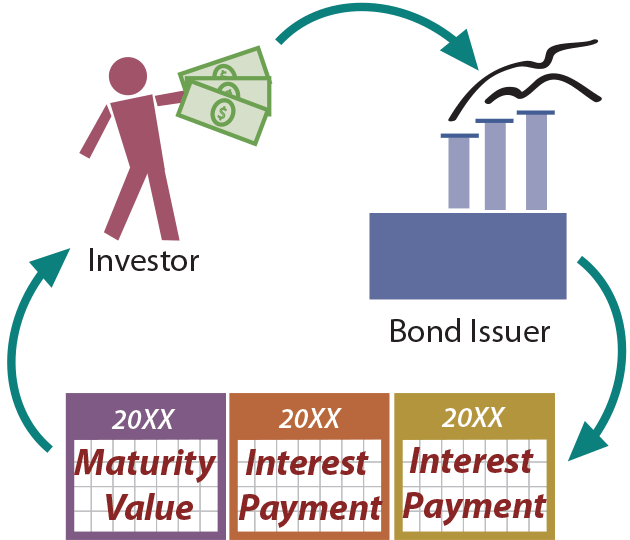It was noted earlier that certain types of financial instruments have a fixed maturity date; the most typical of such instruments are “bonds.” The held-to-maturity securities are normally accounted for by the amortized cost method.

To elaborate, if an individual wishes to borrow money he or she would typically approach a bank or other lender. But, a corporate giant’s borrowing needs may exceed the lending capacity of any single bank or lender. Therefore, the large corporate borrower may instead issue “bonds,” thereby splitting a large loan into many small units.

For example, a bond issuer may borrow \$500,000,000 by issuing 500,000 individual bonds with a face amount of \$1,000 each (500,000 X \$1,000 = \$500,000,000). If an individual wished to loan some money to that corporate giant, he or she could do so by simply buying (“investing in”) one or more of the bonds.

The specifics of bonds will be covered in greater detail in a subsequent chapter, where bonds are examined from the issuer’s perspective (i.e., borrower). For now, bonds will be considered from the investor perspective. Each bond has a “face value” (e.g., \$1,000) that corresponds to the amount of principal to be paid at maturity, a contract or stated interest rate (e.g., 5% — meaning that the bond pays interest each year equal to 5% of the face amount), and a term (e.g., 10 years — meaning the bond matures 10 years from the designated issue date). In other words, a \$1,000, 5%, 10-year bond would pay \$50 per year for 10 years (as interest), and then pay \$1,000 at the stated maturity date.

### The Issue Price

How much would one pay for a 5%, 10-year bond: Exactly \$1,000, more than \$1,000, or less than \$1,000? The answer to this question depends on many factors, including the credit-worthiness of the issuer, the remaining time to maturity, and the overall market conditions. If the “going rate” of interest for other bonds was 8%, one would likely avoid this 5% bond (or, only buy it if it were issued at a deep discount). On the other hand, the 5% rate might look pretty good if the “going rate” was 3% for other similar bonds (in which case one might actually pay a premium to get the bond).

So, bonds might have an issue price that is at face value (also known as par), or above (at a premium) or below (at a discount) face. The price of a bond is typically stated as percentage of face; for example 103 would mean 103% of face, or \$1,030. The specific calculations that are used to determine the price one would pay for a particular bond are revealed in a subsequent chapter.

### Bonds Purchased at Par

An Investment in Bonds account (at the purchase price plus brokerage fees and other incidental acquisition costs) is established at the time of purchase. Premiums and discounts on bond investments are not recorded in separate accounts: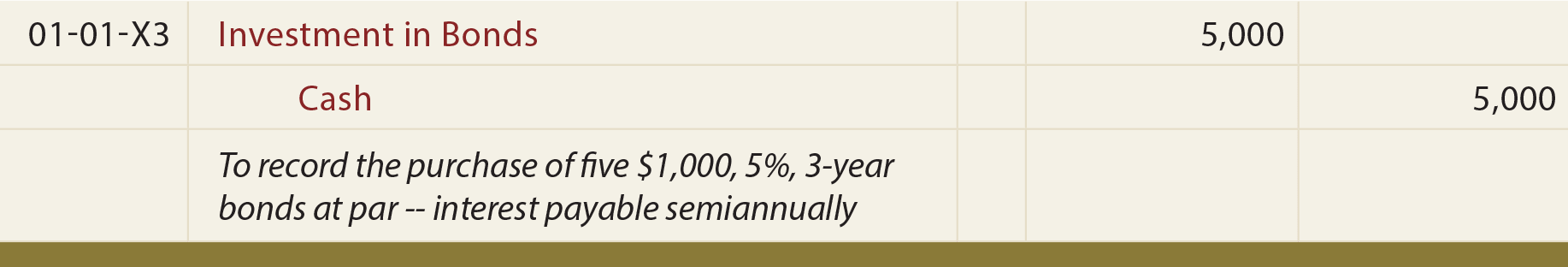The above entry reflects a bond purchase as described, while the following entry reflects the correct accounting for the receipt of the first interest payment after 6 months.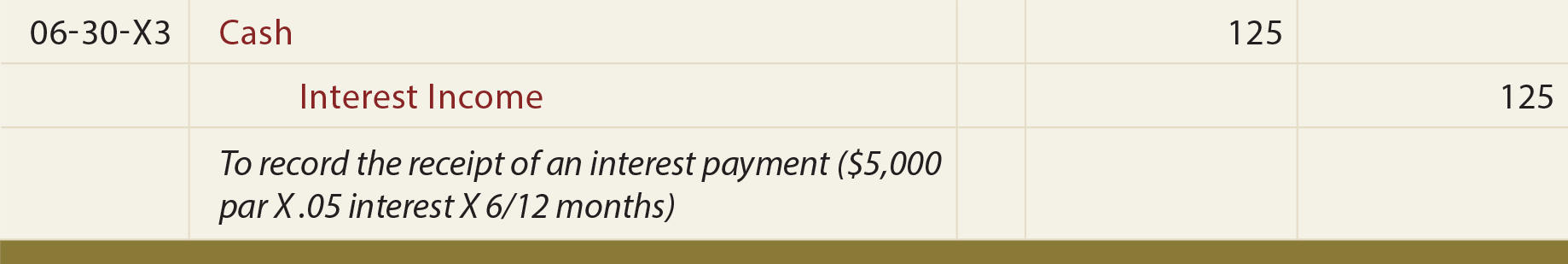The entry that is recorded on June 30 would be repeated with each subsequent interest payment, continuing through the final interest payment on December 31, 20X5. In addition, at maturity, when the bond principal is repaid, the investor would also make this final accounting entry: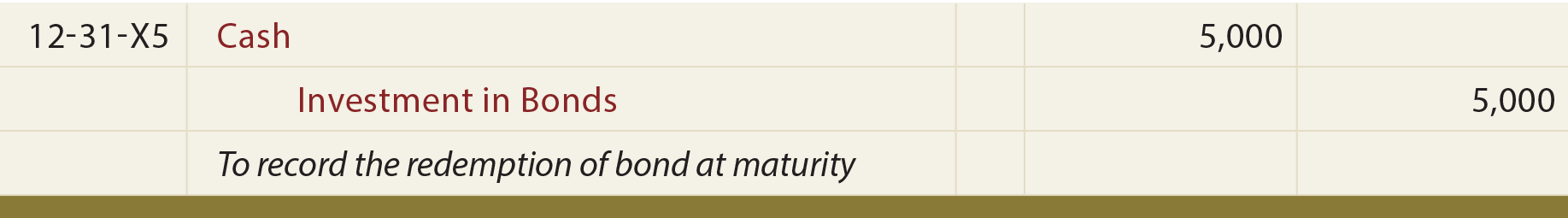### Bonds Purchased at a Premium

When bonds are purchased at a premium, the investor pays more than the face value up front. However, the bond’s maturity value is unchanged; thus, the amount due at maturity is less than the initial issue price! This may seem unfair, but consider that the investor is likely generating higher annual interest receipts than on other available bonds. Assume the same facts as for the preceding bond illustration, but this time imagine that the market rate of interest was something less than 5%. Now, the 5% bonds would be very attractive, and entice investors to pay a premium:The above entry assumes the investor paid 106% of par (\$5,000 X 106% = \$5,300). However, remember that only \$5,000 will be repaid at maturity. Thus, the investor will be “out” \$300 over the life of the bond. Thus, accrual accounting dictates that this \$300 “cost” be amortized (“recognized over the life of the bond”) as a reduction of the interest income: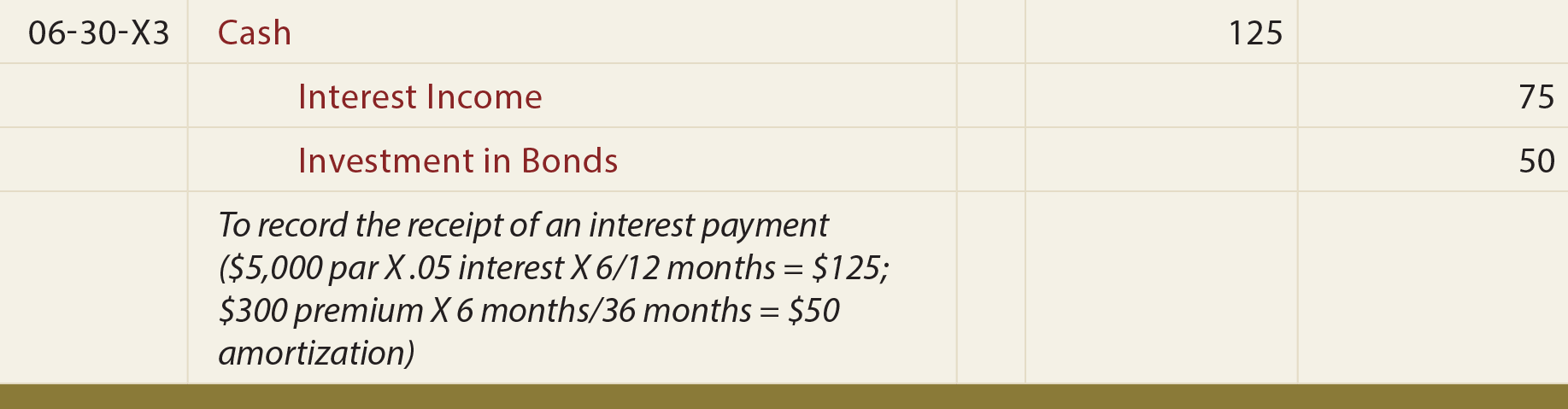The preceding entry can be confusing and bears additional explanation. Even though \$125 was received, only \$75 is being recorded as interest income. The other \$50 is treated as a return of the initial investment; it corresponds to the premium amortization (\$300 premium allocated evenly over the life of the bond: \$300 X (6 months/36 months)). The premium amortization is credited against the Investment in Bonds account. This process of premium amortization would be repeated with each interest payment. Therefore, after three years, the Investment in Bonds account would be reduced to \$5,000 (\$5,300 – (\$50 amortization X 6 semiannual interest recordings)).

This method of tracking amortized cost is called the straight-line method. There is another conceptually superior approach to amortization, called the effective-interest method, which will be revealed in later chapters. However, it is a bit more complex and the straight-line method presented here is acceptable so long as its results are not materially different than would result under the effective-interest method.

In addition, at maturity, when the bond principal is repaid, the investor would make this final accounting entry: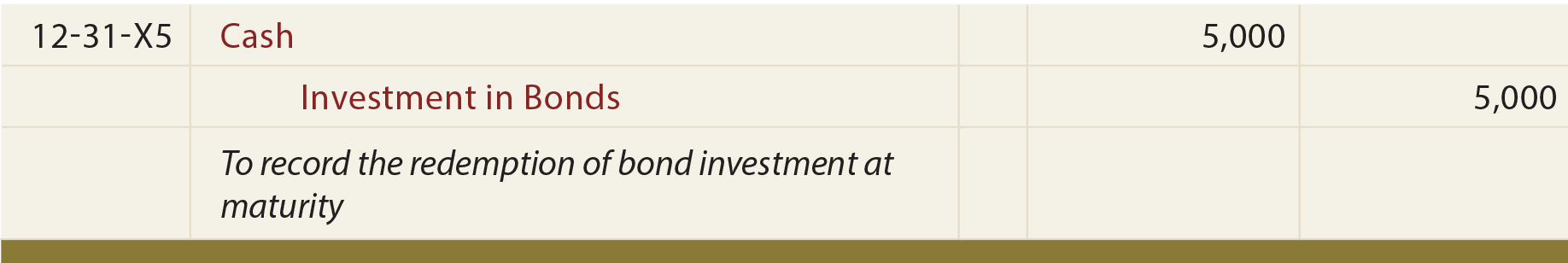In an attempt to make sense of the preceding, perhaps it is helpful to reflect on just the “cash out” and the “cash in.” How much cash did the investor pay out? It was \$5,300; the amount of the initial investment. How much cash did the investor get back? It was \$5,750; \$125 every 6 months for 3 years and \$5,000 at maturity. What is the difference? It is \$450 (\$5,750 – \$5,300). This is equal to the income recognized via the journal entries (\$75 every 6 months, for 3 years). At its very essence, accounting measures the change in money as income. Bond accounting is no exception, although it is sometimes illusive to see. The following “amortization” table reveals certain facts about the bond investment accounting, and is worth studying closely.

Be sure to “tie” the amounts in the table to the illustrated journal entries.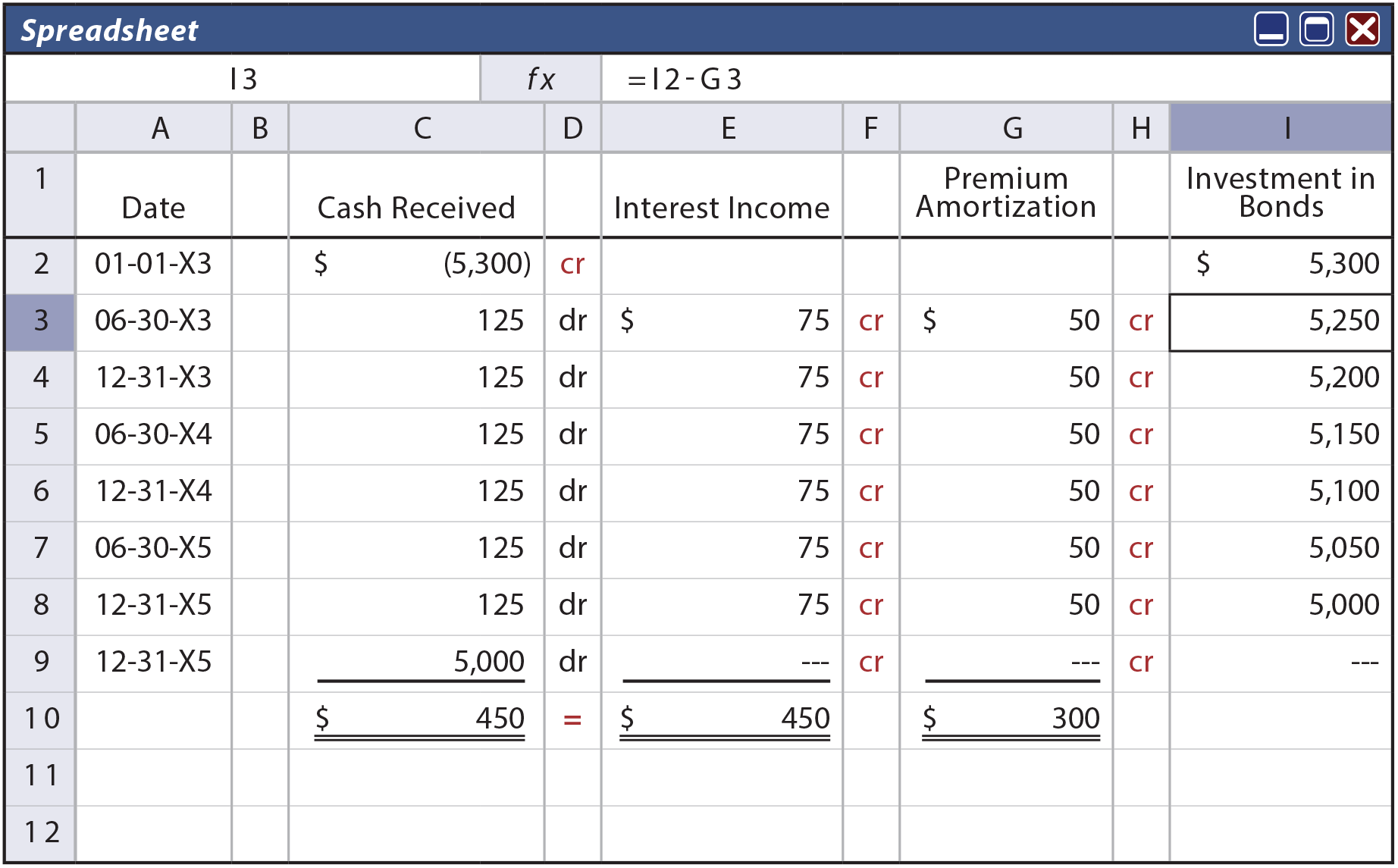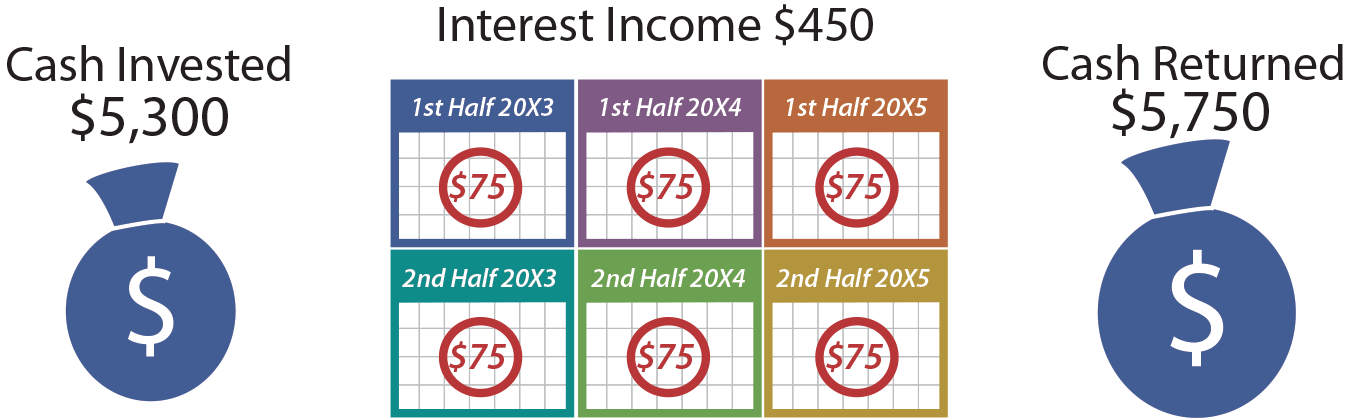Sometimes, complex transactions are easier to understand when one simply thinks about the balance sheet impact. For example, on December 31 20X4, Cash is increased \$125, but the Investment in Bonds account is decreased by \$50 (dropping from \$5,150 to \$5,100). Thus, total assets increased by a net of \$75. The balance sheet remains in balance because the corresponding \$75 of interest income causes a corresponding increase in retained earnings.

### Bonds Purchased at a Discount

The discount scenario is very similar to the premium, but “in reverse.” When bonds are purchased at a discount, the investor pays less than the face value up front. However, the bond’s maturity value is unchanged; thus, the amount due at maturity is more than the initial issue price! This may seem like a bargain, but consider that the investor is likely getting lower annual interest receipts than is available on other bonds.

Assume the same facts as for the previous bond illustration, except imagine that the market rate of interest was something more than 5%. Now, the 5% bonds would not be very attractive, and investors would only be willing to buy them at a discount:The above entry assumes the investor paid 97% of par (\$5,000 X 97% = \$4,850). However, remember that a full \$5,000 will be repaid at maturity. The investor will get an additional \$150 over the life of the bond. Accrual accounting dictates that this \$150 “benefit” be recognized over the life of the bond as an increase in interest income: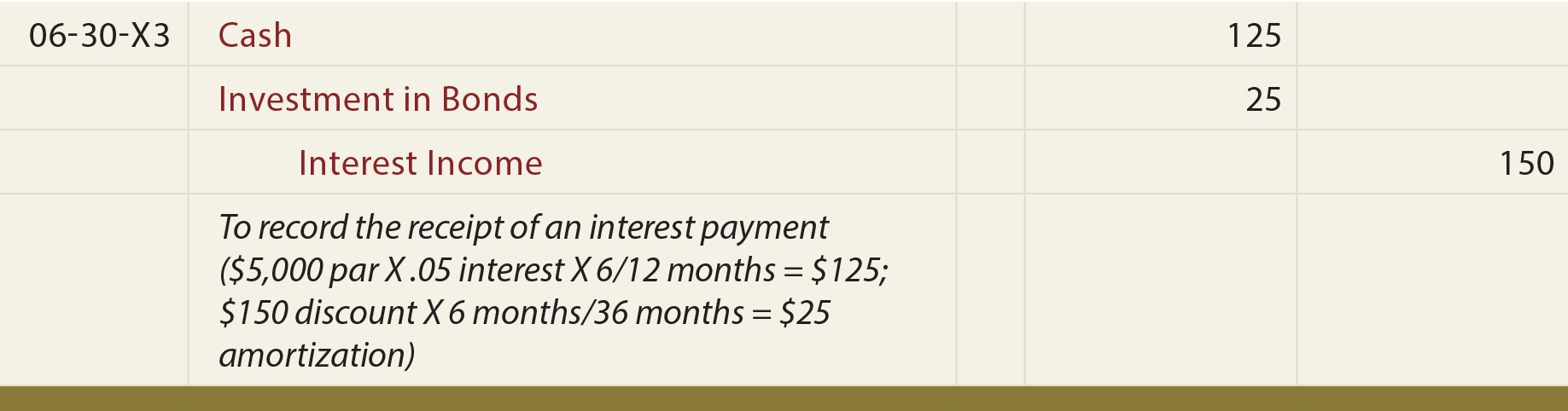The preceding entry would be repeated at each interest payment date. Again, further explanation may prove helpful. In addition to the \$125 received, another \$25 of interest income is recorded. The other \$25 is added to the Investment in Bonds account, as it corresponds to the discount amortization (\$150 discount allocated evenly over the life of the bond: \$150 X (6 months/36 months)=\$25).

This process of discount amortization would be repeated with each interest payment. Therefore, after three years, the Investment in Bonds account would be increased to \$5,000 (\$4,850 + (\$25 amortization X 6 semiannual interest recordings)). This example again uses the straight-line method of amortization since the amount of interest is the same each period. The alternative effective-interest method demonstrated later in the book would be required if the results would be materially different.

When the bond principal is repaid at maturity, the investor would also make this final entry: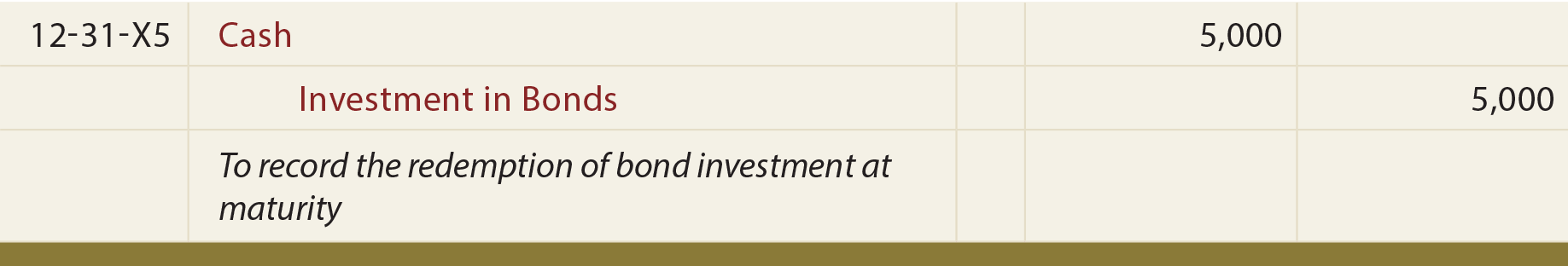Consider the “cash out” and the “cash in.” How much cash did the investor pay out? It was \$4,850; the amount of the initial investment. How much cash did the investor get back? It is the same as it was in the premium illustration: \$5,750 (\$125 every 6 months for 3 years and \$5,000 at maturity). What is the difference? It is \$900 (\$5,750 – \$4,850). This is equal to the income recognized (\$150 every 6 months, for 3 years).

Be sure to “tie” the amounts in the following amortization table to the related entries.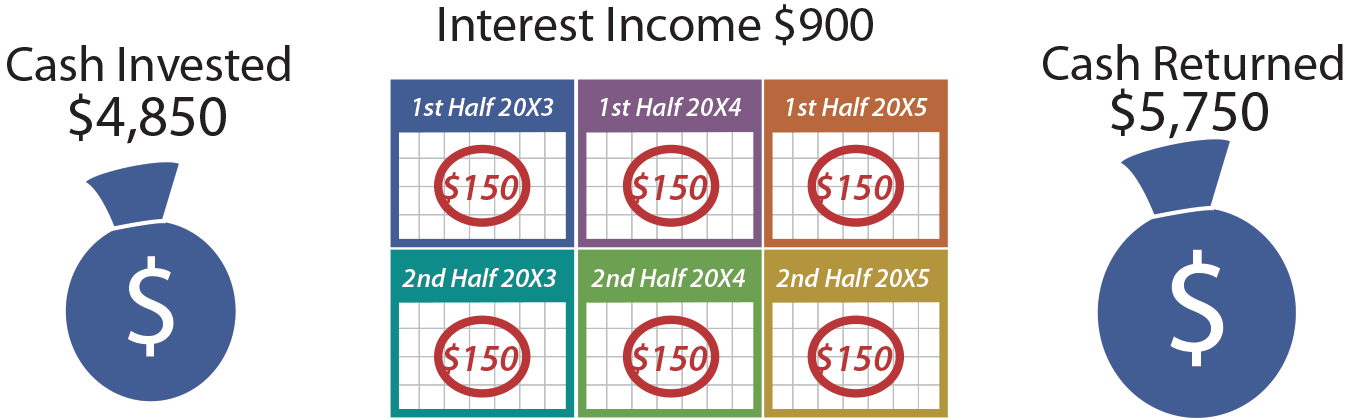What is the balance sheet impact on June 30, 20X5? Cash increased by \$125, and the Investment in Bonds account increased \$25. Thus, total assets increased by \$150. The balance sheet remains in balance because the corresponding \$150 of interest income causes a corresponding increase in retained earnings.

Did you learn?
How should an initial investment in a bond be recorded?
What is meant by the amortized cost method?
Know the meaning of bond terminology, including “issue price,” “face (or par),” “premium,” and “discount.”
Note that the recorded bond investment account includes the amount of premium or discount (a separate account is not used).
Be able to record bond interest income.
Understand why it is necessary to amortize a premium or discount on a bond investment.
Be able to apply the straight-line method of amortization.
Be able to account for the full life cycle of a bond investment, including situations involving premiums and discounts.